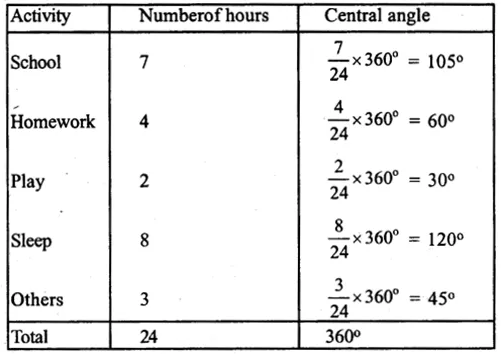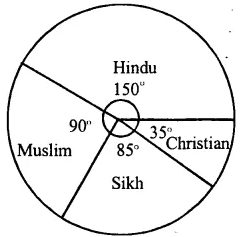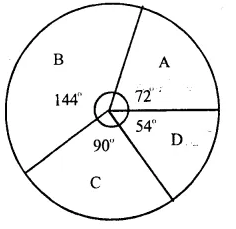# RS Aggarwal Solutions Class 8 Chapter 23 Pie Charts Ex 23A

In this chapter, we provide RS Aggarwal Solutions for Class 8 Chapter 23 Pie Charts Ex 23A for English medium students, Which will very helpful for every student in their exams. Students can download the latest RS Aggarwal Solutions for Class 8 Chapter 23 Pie Charts Ex 23A pdf, free RS Aggarwal Solutions Class 8 Chapter 23 Pie Charts Ex 23A book pdf download. Now you will get step by step solution to each question.

## RS Aggarwal Class 8 Solutions Chapter 23 Pie Charts Ex 23A

Question 1.
Solution:
Total expenditure = Rs. 4000 + 5400 + 2800 + 1800 + 400 = Rs. 14400Construction of pie chart :
1. Draw a circle of any convenient radius.
3. Staring from this radius, draw sectors of central angle 100°, 135°, 70°, 45° and 10° respectively.
4. Shade these sectors with different colors or designs as shown in the figure.
This is the required pie chart.Question 2.
Solution:
Total number of creatures 900(i) Draw a circle with a suitable radius.
(iii) Starting from this radius, draw sectors whose central angles are 60°, 160°, 70°, 50° and 20° respectively.
(iv) Now shade each sector with different colours or designs as shown in the figure.Question 3.
Solution:
Total number of students = 350 + 245 + 210 + 175 + 280 = 1260(i) Draw a circle with a suitable radius.
(iii) Starting from this radius draw sectors whose central angles are 100°, 70°, 60°, 50° and 80° respectively.
(iv) Now shade each sector with different colours or designs as shown in the figure.Question 4.
Solution:(i) Draw a circle with a suitable radius.
(iii) Starting from this radius, draw sectors whose actual angles are 105°, 60°, 30°, 120° and 45° respectively.
(iv) Now shade each sector with different colours or design as shown in the figure.Question 5.
Solution:
Here total number of workers = 1080Now (i) Draw a circle with a suitable radius
(iii) Starting from this radius, draw sectors whose central angle are 150°, 90°, 85°, 35° respectively.
(iv) Now shade the sectors with different colours or designs as shown in the figure.Question 6.
Solution:
Total marks obtained by Sudhir
= 105 + 75 + 150 + 120 + 90 = 540(i) Draw a circle with a suitable radius
(iii) Starting from this radius, draw sectors whose central angles are 70°, 50°, 100°, 80° and 60° respectively
(iv) Now shade these sectors with different colours or designs as shown in the figure.Question 7.
Solution:
Total number of fruits = 26 + 30 + 21 + 5 + 8 = 90(i) Draw a circle with a suitable radius.
(iii) Starting from this radius, draw sectors of central angles 104°, 120°, 84°, 20° and 32° respectively.
(iv) Shade these sectors with different colours or designs as shown in the figure.Question 8.
Solution:
Total number of million of tonnes of food grains = 57 + 76 + 38 + 19 = 190 million of tonnes(i) Draw a circle with suitable radius.
(iii) Starting with this radius, draw sectors of central angles 108°, 144°, 72° and 366 respectively.
(iv) Shade these sectors with different colours or designs as shown in the figure.Question 9.
Solution:
Total percentage = 25 + 45 + 20 + 10 = 100%(i) Draw a circle with a suitable radius.
(iii) Starting from this radius, draw sectors of central angles 90°, 162°, 72° and 36° respectively.
(iv) Shade these sectors with different colours or designs as shown in the figure.Question 10.
Solution:
Total percentage = 20 + 40 + 25 + 15 = 100%(i) Draw a circle with a suitable radius.
(iii) Starting from this radius, draw sectors of central angles 72°, 144°, 90° and 54° respectively.
(iv) Now shade these sectors with different colours or designs as shown in the figure.All Chapter RS Aggarwal Solutions For Class 8 Maths

—————————————————————————–

All Subject NCERT Exemplar Problems Solutions For Class 8

All Subject NCERT Solutions For Class 8

*************************************************

I think you got complete solutions for this chapter. If You have any queries regarding this chapter, please comment on the below section our subject teacher will answer you. We tried our best to give complete solutions so you got good marks in your exam.

If these solutions have helped you, you can also share rsaggarwalsolutions.in to your friends.